# Introduction to Semiconductor Notes for Electronics Engineering B.tech 1st Year

## What is Semiconductor?

The materials that are neither conductor nor insulator with energy gap of about 1 eV (electron volt) are called semiconductors.

A semiconductor is a substance, usually a solid chemical element or compound, that can conduct electricity under some conditions but not others, making it a good medium for the control of electrical current. Its conductance varies depending on the current or voltage applied to a control electrode, or on the intensity of irradiation by infrared (IR), visible light, ultraviolet (UV) or X-rays.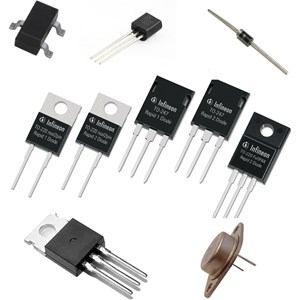## Materials used as Semiconductor:

Most common type of materials that are commercially used as semiconductors are germanium (Ge) and silicon (Si) because of their property to withstand high temperature. That means there will be no significant change in energy gap with changing temperature. The relation between energy gap and absolute temperature for Si and Ge are given as,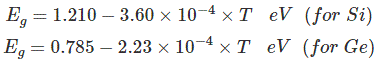Where, T = absolute temperature in oK

Assuming room temperature to be 300oK,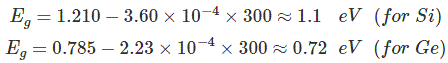At room temperature resistivity of semiconductor is in between insulators and conductors. Semiconductors show negative temperature coefficient of resistivity that means its resistance decreases with increase in temperature. Both Si and Ge are elements of IV group i.e. both elements have 4 valence electrons. Both form the covalent bond with the neighboring atom. At absolute zero temperature both behave as insulator i.e. the valence band is full while conduction band is empty but as the temperature is raised more and more covalent bonds break and electrons are set free and jump to the conduction band.

In the above energy band diagrams of a semiconductor. CB is the conduction band and VB is the valence band. At 0°K, the VB is full with all the valence electrons.

## 1.Intrinsic Semiconductors:

As per theory of semiconductor, semiconductor in its pure form is called as intrinsic semiconductor. In pure semiconductor number of electrons (n) is equal to number of holes (p) and thus conductivity is very low as valence electrons are covalent bonded. In this case we write n = p = ni, where ni is called the intrinsic concentration. It can be shown that ni can be written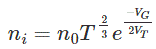Where, n0 is a constant, T is the absolute temperature, VG is the semiconductor band gap voltage, and VT is the thermal voltage.

## 2.Extrinsic Semiconductors:

As per theory of semiconductor, impure semiconductors are called extrinsic semiconductors. Extrinsic semiconductor is formed by adding a small amount of impurity. Depending on the type of impurity added we have two types of semiconductors: N – type and P-type semiconductors. In 100 million parts of semiconductor one part of impurity is added.

## N-type Semiconductor:

In this type of semiconductor majority carriers are electrons and minority carriers are holes. N – type semiconductor is formed by adding pentavalent (five valence electrons) impurity in pure semiconductor crystal, e.g. P. As, Sb.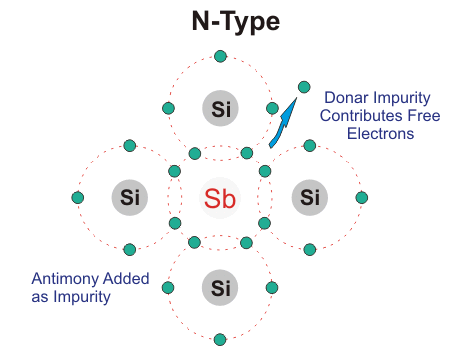Four of the five valence electron of pentavalent impurity forms covalent bond with Si atom and the remaining electron is free to move anywhere within the crystal.

## P-type Semiconductors:

In this type of semiconductor majority carriers are holes and minority carriers are electrons. pure semiconductor crystal, e.g. B, Al Ba.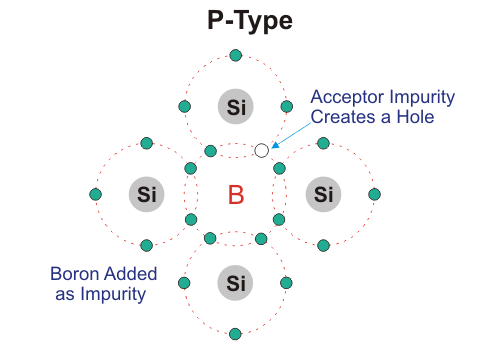Three of the four valence electron of tetravalent impurity forms covalent bond with Si atom. This leaves an empty space which is referred to as hole. When temperature is raised electron from another covalent bond jumps to fill this empty space. This leaves a hole behind. In this way conduction takes place. P- type impurity accepts electron and is called acceptor atom. Majority carriers are holes and therefore conductivity is due to these holes only and is given by, σ= neμh

### Feedback is important to us.

error: Content is protected !!